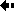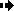## Genetic loadGenetic load is a number between 0 and 1 and it measures the extent to which the average individual in a population is inferior to the best possible kind of individual. The genetic load equals the relative chance that an average individual will die before reproducing because of the deleterious genes that it possesses. Ignoring frequency-dependent selection, it is calculated as follows:

Suppose there are a variety of genotypes in the population, each with its characteristic fitness; one genotype has a higher fitness than the rest and we call its fitness Wopt. We can also measure the average fitness of the whole population; it is just the fitness of each genotype multiplied by its frequency: it is called mean fitness and is symbolised by v.

The general formula for genetic load (L) is as follows:

L = (Wopt - v) / Wopt

If all the individuals in the population have the optimal genotype, then v = Wopt and the load is zero. If all but one have a genotype of zero fitness then v = 0 and L = 1.

Genetic load can exist for several reasons, of which mutational load is the most important.Previous Next